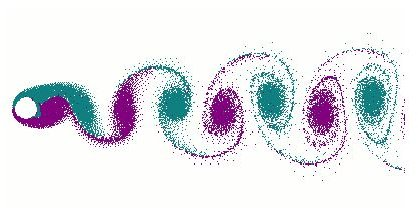# Vortex Shedding Frequency Equation and Determining the Vortex Shedding

When fluid flows over any object a region of velocity variation among the layer of the fluid develops. This region is known as a boundary layer. However, it consists of different regions; they are the laminar region, transition region, and turbulent region. The fluid moves very smoothly up to the transition region. Special turbulent conditions are the cause of vortex shedding. If a body has rounded shape or blunt structure the chances of vortex shedding will be elevated. A common example is mixing sugar in a cup of tea using a spoon. We develop vortex shedding in the fluid with the help of spoon mixing a certain quantity of sugar. Because of this phenomenon, you mix the sugar easily. The whole process will become much more difficult if you attempt to move the cup and keep the spoon immobile instead.

## The Importance of Prediction of Vertex Shedding

Determining the amount of vortex shedding is very important especially in the construction of tall structures like smoke stack chimneys. When highspeed wind flows towards these chimneys vortex shedding occurs. This is a region of very low pressure that forces the chimney towards it. If the vortices that shed off the chimney have a frequency equal to the resonance frequency of the chimney then it starts swaying out of control. This causes the destruction of the structure. Due to this, prediction of vertex shedding is essential. Methods of restricting its formation are also used, such as putting obstructions along the chimney.

However, predicting vortex shedding is complicated in hydraulics or hydrodynamics. It may occur in areas like cooling tower and water condensate, municipal water treatment and distribution, chemical processing, boiler feed water, water management, pool and Water Park etc.

## How do we predict Vortex Shedding?

The prediction of vortex shedding is only possible by mathematical calculations. It can be predicted by the non-dimensional Strohal Number. This number is also expressed as ‘St’, and the equation is known as vortex shedding frequency equation. The formula of finding the value of St Number is-

St= f*v/L

Where,

‘f’ is the frequency of shedding vortices,

L is the characteristic length and, and

V is the velocity of the flow.

The value of St Number provides the indication of occurrence of vortex shedding, mathematically. It must have the value in between 0.0001 and 1 for the formation of vortex shedding.

If the value of St number lies in between the given value, the shear speed of the layers of the fluid start dissipating vortex shedding. However, if the St number is too high, the effect on the viscosity of the fluid will be high; due to this, the frictional force between the layers start opposing the formation of sheds.

## Finding the Value of Frequency (f)

This is the value of frequency of the fluctuation of the fluid taking place at the downside of the object. You can predict the frequency of the object using a Vortex Flowmeter. You will need to apply this flowmeter to the pipe and it will calculate the frequency of the vortex shedding automatically. They are available in the market in various sizes in terms of diameter. You can choose them as per the requirement.

This instrument provides the output in digital form with good accuracy. It can be used over a wide flow range. It has no moving parts, and hence it offers long-term stability and repeatability.

## Finding the Value of Fluid Velocity (v)

Finding the velocity of the fluid is very easy. It requires using very common instruments in hydraulics such as velocity flowmeter, ultrasonic doppler flowmeter. Orifice plate, venturi tube, and flow nozzles can also be used to determine the velocity of the fluid.

## Finding the Effective Length (L)

The measurement of effective length is different for different shapes. However, for the fluid flowing through pipes, the effective length will be the diameter of the pipe.

## Conclusion

After finding the required measurements, i.e., velocity of the fluid, frequency of the vortex shedding, and the effective length, calculate the value of the St number. If the value of the St number will be in between 0.0001 and1, the vortex shedding will start. This is how the vortex shedding frequency equation is used to predict the start of the process.

## References

Vortex Shedding Phenomena explored

Introduction to Vortex Flowmeters

Image – Vortex Shedding- courtesy of Cesareo de La Rosa Siqueira